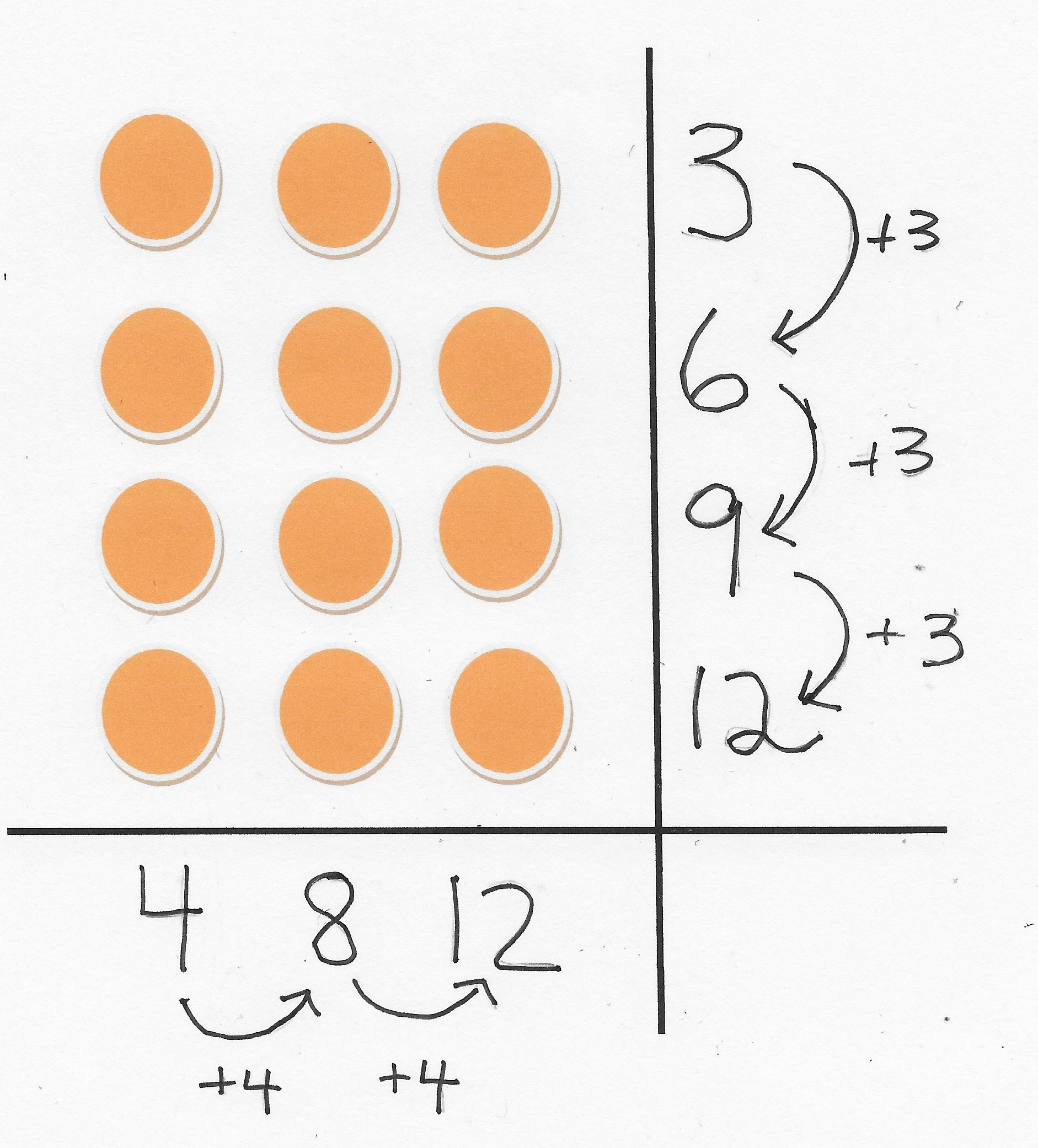# Trading Cards and Multiplication Tables

### PROBLEM: If you place, on an empty table top, five rows of trading cards with three in each row, then how many cards will be on the table?

This problem exemplifies Power-Start Goal #3 of knowing from memory the one-digit multiplication facts and Power-Start Goal #4 use operations (here multiplication) to solve problems.  Knowing the “multiplication tables” is a super-important third-grade accomplishment, one which prepares the way for math proficiency at higher levels of schooling.   Today, many students leave third grade without committing these basic facts to memory, but the good news is that this accomplishment can be greatly facilitated by giving preschool children experiences envisioning multiplication situations with small numbers, such as 3 and 3 is two groups of 3 which totals 6 things.  These experiences introduce children to multiplicative thinking much earlier and prepare them for understanding and learning larger facts like 7 times 9 is 63 by the end of third grade.

### Multiplicative Thinking before Memorizing

Multiplicative thinking entails basic understandings of combining a certain number of same-sized groups to determine a total number;  and also the idea of dividing a total number of things into equal groups or fair shares. The proficient third-grader will read this word problem about trading cards and know that multiplication is the operation to use and then quickly produce the correct answer of 15 trading cards.  Here are a few related ideas which multiplicative thinking will connect to the fact 3 x 5 = 15.

• 5 x 3 = 3 x 5 = 15 (commutative property of multiplication)
• 15 ÷ 3 = 5 and 15 ÷ 5 = 3
• 15 is a multiple of both 5 and 3
• Both 3 and 5 are factors of 15.
• When the minute hand on the clock moves past 1 and 2 and on to 3, then 5, 10, 15 minutes have elapsed.
• 3 five-dollar bills make a total of \$15.

## First steps on a multiplication learning trajectory…

Two ways to introduce multiplicative thinking to preschoolers involve skip counting and for hands-on experiences with small-number row-and-column arrays.

### Skip-Counting

The term “skip counting” describes processes of moving ahead in number order more quickly.   Counting by twos – 2,4,6,8,… might be introduced by counting shoes.  Counting by tens – 10, 20, 30, 40,… 100 is a quick way for counting to 100 and a starting place for understanding our number system’s base-ten structure.     We can build knowledge and understanding of 3 x 5 = 15 with skip-counting by 3 and by 5.  You can practice skip counting with children riding in the car or any time.  Try out these skip-counting videos on YouTube.

### Row-and-Column Arrays

In addition to skip-counting, making arrays of rows and columns is another good way to begin early with multiplicative thinking. Our children today look at screens much more than we did as children and their visual abilities are superb.  So, when opportunities present themselves, use your math lenses to connect arrays of objects with multiplication situations. In the photo, the boy is pointing to a rectangular array of 6 poker chips, 2 rows and 3 in each row. The girl is pointing to a 3 by 3 square array with 9 poker chips in all.   In talking about these arrays, cover up rows and columns and talk about how many you see and how many are hidden. Say aloud related addition and subtraction facts, such as “3 and 3 is 6” and “9 take away 3 is 6.”

For additional ideas about linking the row-and-column concept with one-digit multiplication facts go to my page,“Excel for Prekindergarten” ,under the “Activities” tab.

### Arrays, Skip Counting, and Repeated Addition with the Number 12

Twelve is a special number for many reasons: 12 numbers on the face of an analog clock; the number of spaces in a carton holding a dozen eggs.  Twelve is also a number that has more factors than most numbers less than 20.  Factors of 12 are 1 and 12; 2 and 6; 3 and 4.     The diagram here pictures an array of 4 rows with 3 in each row, and the diagram also shows how the number 12 can be understood in terms of  skip counting (3, 6, 9, 12) and repeated addition

• 3 + 3 = 6
• 6+ 3 =9
• 9 + 3 = 12

The boy’s white array of poker chips with 2 rows of 3 and the girl’s blue array of 3 rows of 3 can be used to build the array in the diagram.    Here are a few other ideas for zooming in with your math lenses on the number 12

• Cover up rows and columns of a 3 by 4 array and relate what you see and don’t see to addition and subtraction facts.
• Count by 3s to 12 while making a complete circle around the face of an analog clock (3 — quarter hour; 6 — half hour; 9 — three-quarters hour; 12 — one whole hour has passed)
• Rearrange the array as 2 rows of 6 and then place the poker chips in an egg carton — one space for each chip.

### NEXT WEEK’S POST: Multiplication Tables and Mathematical Rigor

We will move from a problem focusing on a multiplication fact with product less than 20 (5 x 3 = 15) to the more-difficult-to-remember fact of 7 x 9 = 63.